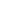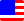Documents  >  Reference  >  Expressions
Expressions in the Rice

Expressions

The expression is an element that returns the address of the instance. The combination of the expression and operator will constitute a new expression.

int i = 45;

The literal of the right side is an expression that returns the address of the int type instance with a value of 45.

int j = i + 55;

The arithmetic expression of the right side is an expression that returns the address of the int type instance with a value of 100. This arithmetic expression is made of the combining of variable reference expression, addition operator, and literal expression.

Operators

Operators are a symbol to specify the operation.

List and priorities

When an expression contains multiple operators, the order of evaluation of the expression is determined by the precedence of the operators. When operators with the same precedence are lined up, the evaluation order is determined by associativity of the operators.

The following is the list of the expressions, and its priority. The lower number is the high priority.

 1 () x(y) method call operator new new int() new operator this this this operator [] x[y] indexer operator 2 . x.y dot operator ++ x++ postfix ++ operator -- x-- postfix -- operator 3 + +x unary + operator - -x unary - operator ! !x logical negation operator ++ ++x prefix ++ operator -- --x prefix -- operator 4 * x * y multiplication operator / x / y division operator % x % y modulo operator 5 + x + y addition operator - x - y subtraction operator 6 < x < y less than operator <= x <= y less than or equal operator > x > y greater than operator >= x >= y greater than or equal operator 7 == x == y value equality operator != x != y value inequality operator \$\$ x \$\$ y reference equality operator !\$ x !\$ y reference inequality operator 8 & x & y logical AND operator 9 | x | y logical OR operator

Changing the evaluation order

Expression that enclosed by parentheses is evaluated with priority.

method call operator

The method call operator run the specified code with the method name and argument. The argument is zero or more expressions separated by commas in parentheses. It is passed to the method at the start of execution.

new operator

The new operator run the specified fitter with the class name and argument, and return an address of the new instance. The argument is zero or more expressions separated by commas in parentheses. It is passed to the fitter at the start of execution.

this operator

The this operator refers to the current instance of the class.

indexer operator

Access items of the list class. The instance returned by the expression on the left side must be a list. The instance returned by the expression between [] must be an int.

Returns the address of the item determined by the expression between [] from the referenced list class instance on the left side of the operator.

If the operand returns a proxy class, the entity of the proxy is used.

dot operator

The dot operator provide to member access of the instance returned by the expression of left side.

postfix ++ operator

Returns the address of a new instance that has the same value of the left side expression of the operator and increments the value of the left side expression. The instance returned by the left side expression must be int, long, real.

If the operand returns a proxy class, the entity of the proxy is used.

postfix -- operator

Returns the address of a new instance that has the same value of the left side expression of the operator and decrements the value of the left side expression. The instance returned by the left side expression must be int, long, real.

If the operand returns a proxy class, the entity of the proxy is used.

unary + operator

The unary + operator returns an address of the new instance that has an same value. The type of the instance that is returned by the right side expression must be the int, long, or real.

If the operand returns a proxy class, the entity of the proxy is used.

unary - operator

The unary - operator returns an address of the new instance that has an inverted sign. The type of the instance that is returned by the right side expression must be the int, long, or real.

If the operand returns a proxy class, the entity of the proxy is used.

logical negation operator

The logical negation operator returns an address of the new instance that has an inverted boolean value. The type of the instance that is returned by the right side expression must be the bool.

If the operand returns a proxy class, the entity of the proxy is used.

prefix ++ operator

Increments the value of the right side expression of the operator and returns the address of the new instance that has that value. The instance returned by the right side expression must be int, long, real.

If the operand returns a proxy class, the entity of the proxy is used.

prefix -- operator

Decrements the value of the right side expression of the operator and returns the address of the new instance that has that value. The instance returned by the right side expression must be int, long, real.

If the operand returns a proxy class, the entity of the proxy is used.

arithmetic operation

Binary operators "* /% + -" are called as the arithmetic operators. Type of both sides of the operators must be the int, long, or real.

Exceptionally, the implicit type conversion is carried out in arithmetic operations. Namely, the int, long, and real type will allow to mix in arithmetic operations.

A result of the operation depends on the type of operands. The result will be a real if there is a real in the operands. The result will be a long if there is not a real and there is a long. The other result will be the int.

multiplication operator

The multiplication operator returns an address of the new instance that has the multiplied value of the operand. When the result value is out of the range of result type, an exception will be thrown.

If the operand returns a proxy class, the entity of the proxy is used.

division operator

The division operator returns an address of the new instance that has the division value of the operand. When the result value is out of the range of result type, an exception will be thrown.

If the operand returns a proxy class, the entity of the proxy is used.

modulo operator

The modulo operator returns an address of the new instance that has the remainder value of the operand. When the result value is out of the range of result type, an exception will be thrown.

If the operand returns a proxy class, the entity of the proxy is used.

The addition operator returns an address of the new instance that has the addition value of the operand. When the result value is out of the range of result type, an exception will be thrown.

If the operand returns a proxy class, the entity of the proxy is used.

subtraction operator

The subtraction operator returns an address of the new instance that has the subtraction value of the operand. When the result value is out of the range of result type, an exception will be thrown.

If the operand returns a proxy class, the entity of the proxy is used.

concatenation operator

When the type of both sides operand is the string, binary operators + is called as the concatenation operator. Concatenation operator returns the address of the new instance of string type that is the concatenation of operands.

If the operand returns a proxy class, the entity of the proxy is used.

relational operator

Binary operators "<,<=,>,>=" are called as the relational operators. The operand type of the relational operator must be int, long or real. These can be mixed in relational operation. A result type of the operation is the bool.

less than operator

If the left side operand is less than the right side, the operator returns true. Otherwise returns false.

If the operand returns a proxy class, the entity of the proxy is used.

less than or equal operator

If the left side operand is less than or equal to the right side, the operator returns true. Otherwise returns false.

If the operand returns a proxy class, the entity of the proxy is used.

greater than operator

If the left side operand is greater than the right side, the operator returns true. Otherwise returns false.

If the operand returns a proxy class, the entity of the proxy is used.

greater than or equal operator

If the left side operand is greater than or equal to the right side, the operator returns true. Otherwise returns false.

If the operand returns a proxy class, the entity of the proxy is used.

value comparison operator

Binary operators "==,!=" are called as the value comparison operators. When the operand type of the value comparison operator is string or bool, the types of the left and right operands must be the same. When the operand type is int, long or real, they can be mixed in the value comparison operation. A result type of the operation is the bool.

value equality operator

If the value of left side operand is the same as right side, the operator returns true. Otherwise returns false.

If the operand returns a proxy class, the entity of the proxy is used.

value inequality operator

If the value of left side operand is different from right side, the operator returns true. Otherwise returns false.

If the operand returns a proxy class, the entity of the proxy is used.

reference comparison operator

Binary operators "\$\$,!\$" are called as the reference comparison operators. There is no limit to the type of operands. A result type of the operation is the bool.

reference equality operator

If the left side operand refer the same instance as right side, the operator returns true. Otherwise returns false.

reference inequality operator

If the left side operand do not refer the same instance as right side, the operator returns true. Otherwise returns false.

logical operator

Binary operators "&,|" are called as the logical operators. The type of operands of the logical operator must be the bool. A result type of the operation is the bool.

logical AND operator

If the both operands are the true, the operator returns true. Otherwise returns false.

If the operand returns a proxy class, the entity of the proxy is used.

logical OR operator

If the either operand is the true, the operator returns true. Otherwise returns false.

If the operand returns a proxy class, the entity of the proxy is used.